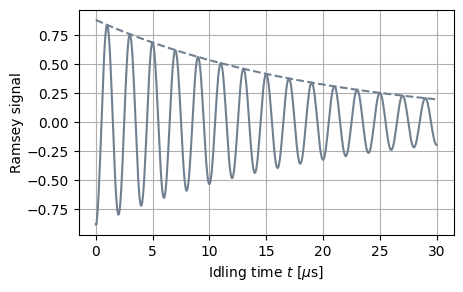# measuring the relaxation time with the idling gate¶

In order to demonstrate the simulation of decoherence noise, we build an example that simulates a Ramsey experiment as a quantum circuit run on a noisy Processor. The Ramsey experiment consists of a qubit that is initialized in the excited state, undergoes a $\pi/2$ rotation around the $x$ axis, idles for a time $t$, and is finally measured after another $\pi/2$ rotation:

In :
import matplotlib.pyplot as plt
import numpy as np
import scipy
from qutip import sigmaz, basis, sigmax, fidelity
from qutip_qip.pulse import Pulse
from qutip_qip.device import LinearSpinChain
from qutip_qip.circuit import QubitCircuit
pi = np.pi
num_samples = 500
amp = 0.1
f = 0.5
t2 = 10 / f

# Define a processor.
proc = LinearSpinChain(
num_qubits=1, sx=amp/2, t2=t2)
ham_idle = 2*pi * sigmaz()/2 * f
resonant_sx = 2*pi * sigmax() - \
ham_idle / (amp/2)
resonant_sx, targets=0, label="sx0")

# Define a Ramsey experiment.
def ramsey(t, proc):
qc = QubitCircuit(1)
result = proc.run_state(
init_state=basis(2, 0),
e_ops = sigmaz()
)
return result.expect[-1]

idle_tlist = np.linspace(0., 30., num_samples)
measurements = np.asarray([ramsey(t, proc) for t in idle_tlist])

rx_gate_time = 1/4/amp # pi/2
total_time = 2*rx_gate_time + idle_tlist[-1]
tlist = np.linspace(0., total_time, num_samples)

peak_ind = scipy.signal.find_peaks(measurements)
decay_func = lambda t, t2, f0: f0 * np.exp(-1./t2 * t)
(t2_fit, f0_fit), _ = scipy.optimize.curve_fit(decay_func, idle_tlist[peak_ind], measurements[peak_ind])
print("T2:", t2)
print("Fitted T2:", t2_fit)

fig, ax = plt.subplots(figsize = (5, 3), dpi=100)
ax.plot(idle_tlist[:], measurements[:], '-', label="Simulation", color="slategray")
ax.plot(idle_tlist, decay_func(idle_tlist, t2_fit, f0_fit), '--', label="Theory", color="slategray")
ax.set_xlabel(r"Idling time $t$ [$\mu$s]")
ax.set_ylim((ax.get_ylim(), ax.get_ylim()))
ax.set_position([0.18, 0.2, 0.75, 0.75])
ax.grid()

T2: 20.0
Fitted T2: 20.05671956607771In the above block, we use the linear spin chain processor just for its compiler and do not use any of its default Hamiltonians. Instead, we define an always-on drift Hamiltonian $\sigma^z$ with frequency $f=0.5$MHz, an on-resonant $\sigma^x$ drive with an amplitude of $0.1/2$MHz and the coherence time $T_2=10/f$. For different idling time $t$, we record the expectation value with respect to the observable $\sigma^z$ as the solid curve. As expected, the envelope follows an exponential decay characterized by $T_2$ (dashed curve). Notice that, because $\pi/2$-pulses are simulated as a physical process, the fitted decay does not start from 1. This demonstrates a way to include state preparation error into the simulation.

In :
import qutip_qip
print("qutip-qip version:", qutip_qip.version.version)
from qutip.ipynbtools import version_table
version_table()

qutip-qip version: 0.2.0

Out:
SoftwareVersion
QuTiP4.6.3
Numpy1.22.2
SciPy1.8.0
matplotlib3.5.1
Cython0.29.27
Number of CPUs12
BLAS InfoOPENBLAS
IPython8.0.1
Python3.9.0 | packaged by conda-forge | (default, Nov 26 2020, 07:53:15) [MSC v.1916 64 bit (AMD64)]
OSnt [win32]
Sun Feb 13 12:25:17 2022 W. Europe Standard Time